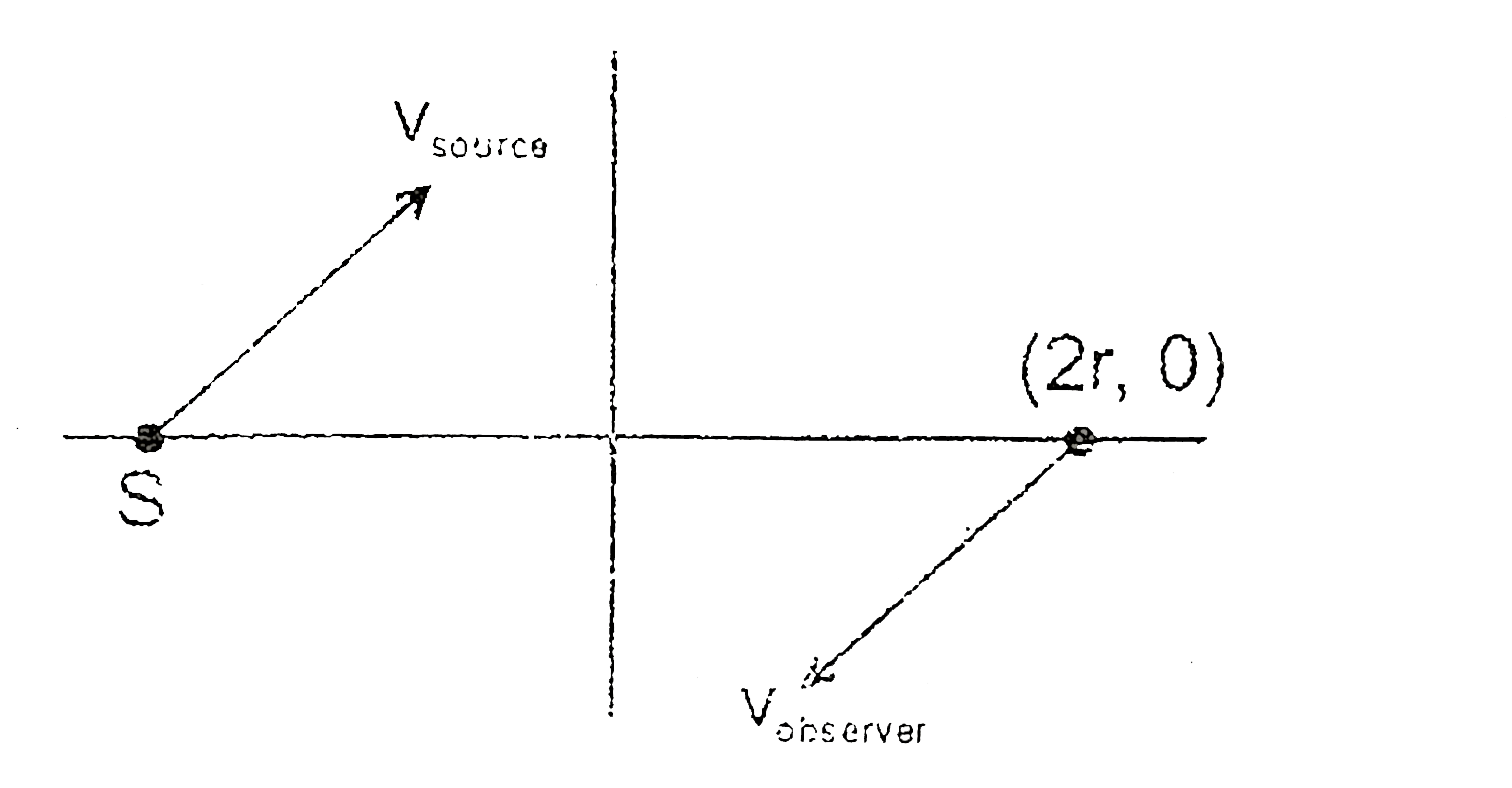# A source which is emitting sound of frequency f is initially at (-r,0) and an observer is situated initally at (2r, 0). If observer and source b

225 views
in Physics
closed
A source which is emitting sound of frequency f is initially at (-r,0) and an observer is situated initally at (2r, 0). If observer and source both are moving with velocities overset(vec)(v)_("observer") = -sqrt(2)Vhat(i) - sqrt(2)hat (j) and overset(vec)(v)_("source") = (V)/(sqrt(2))hat(i) + (V)/(sqrt(2))hat(j), then which of the following is correct option ?
A. Apparent frequency first increases, then decreases and observer the original frequency once during the motion.
B. Apparent frequency first increases, then decreases and observer the original frequency twice during the motion.
C. Apparent frequency first increases, then decreases during the motion and observer never observer the initial frequency.
D. Apparent frequency continously decreases and one during the motion, observer hears the original frequency.

by (85.3k points)
selectedvelocity of approach of observer & source decreases, becomes zero and finally becomes velocity of separation.
Hence apparent frequnency continuously decreases. (f_(app = f when v_(app) = 0)